SLVS681F June   2006  – August 2014

PRODUCTION DATA.

1. Features
2. Applications
3. Description
4. Revision History
5. Device Options
6. Pin Configuration and Functions
7. Specifications
8. Detailed Description
1. 8.1 Overview
2. 8.2 Functional Block Diagram
3. 8.3 Feature Description
4. 8.4 Device Functional Modes
5. 8.5 Programming
1. 8.5.1 EasyScale: One-Pin Serial Interface for Dynamic Output Voltage Adjustment
1. 8.5.1.1 General
2. 8.5.1.2 Protocol
9. Application and Implementation
1. 9.1 Application Information
2. 9.2 Typical Applications
1. 9.2.1 TPS6240x, Dual Outputs Step Down Converter
2. 9.2.2 Various Output Voltages
3. 9.2.3 Dynamic Voltage Scaling on Converter 1 by DEF_1 Pin
10. 10Power Supply Recommendations
11. 11Layout
12. 12Device and Documentation Support
13. 13Mechanical, Packaging, and Orderable Information

• DRC|10
• DRC|10

## 9 Application and Implementation

### 9.1 Application Information

The TPS6240x family of devices are synchronous dual step-down DC-DC converters. The devices provide two independent output voltage rails. The following information gives guidance on choosing external components to complete the application design.

### 9.2 Typical Applications

#### 9.2.1 TPS6240x, Dual Outputs Step Down Converter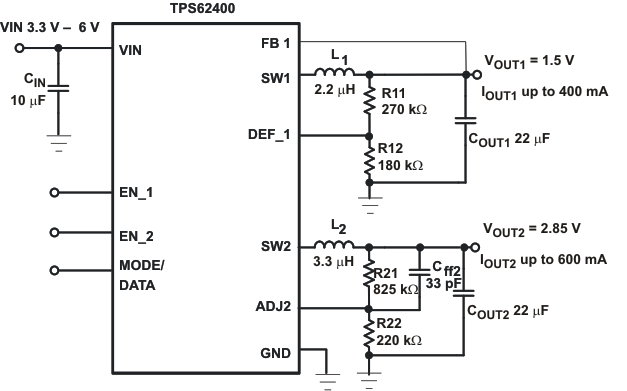Figure 14. Typical Application Circuit 1.5V/2.85V Adjustable Outputs, Low PFM Voltage Ripple Optimized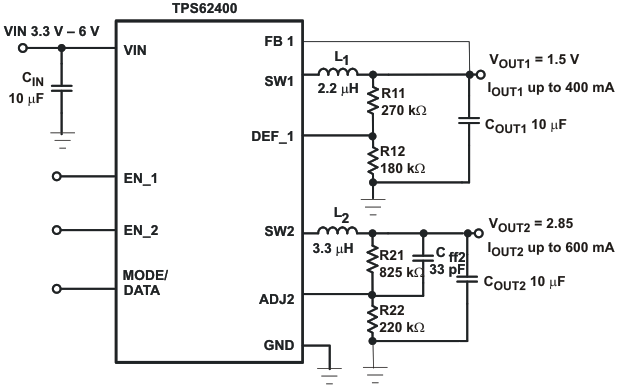Figure 15. Typical Application Circuit 1.5V/2.85V Adjustable Outputs

#### 9.2.1.1 Design Requirements

The step-down converter design can be adapted to different output voltage and load current needs by choosing external components appropriate. The following design procedure is adequate for whole VIN, VOUT and load current range of TPS6240x.

#### 9.2.1.2.1 Converter1 Adjustable Default Output Voltage Setting: TPS62400

The output voltage can be calculated to:

Equation 4.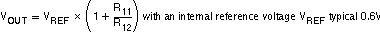To keep the operating current to a minimum, it is recommended to select R12 within a range of 180kΩ to 360kΩ. The sum of R12 and R11 should not exceed ~1MΩ. For higher output voltages than 3.3V, it is recommended to choose lower values than 180kΩ for R12. Route the DEF_1 line away from noise sources, such as the inductor or the SW1 line. The FB1 line needs to be directly connected to the output capacitor. A feed-forward capacitor is not necessary.

#### 9.2.1.2.2 Converter1 Fixed Default Output Voltage Setting (TPS62401, TPS62402, TPS62403, TPS62404).

The output voltage VOUT1 is selected with DEF_1 pin.

Pin DEF_1 = low:

TPS62401, TPS62403 = 1.575V

TPS62402, TPS62404 = 1.2V

Pin DEF_1 = high:

TS62401, TPS62403 = 1.1V

T62402: = 1.8V

T62404: = 1.9V

#### 9.2.1.2.3 Converter 2 Adjustable Default Output Voltage Setting TPS62400:

The output voltage of converter 2 can be set by an external resistor network. For converter 2 the same recommendations apply as for converter1. In addition to that, a 33pF feed-forward Capacitor Cff2 for good load transient response should be used. The output voltage can be calculated to:

Equation 5.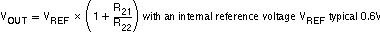#### 9.2.1.2.4 Converter 2 Fixed Default Output Voltage Setting

ADJ2 pin must be directly connected with VOUT2

TPS62401, VOUT2 default = 1.8V

TPS62403, VOUT2 default = 2.8V

TPS62402, TPS62404, VOUT2 default = 3.3V

#### 9.2.1.2.5 Output Filter Design (Inductor and Output Capacitor)

The converters are designed to operate with a minimum inductance of 1.75μH and minimum capacitance of 6μF. The device is optimized to operate with inductors of 2.2μH to 4.7μH and output capacitors of 10μF to 22μF.

#### 9.2.1.2.5.1 Inductor Selection

The selected inductor has to be rated for its DC resistance and saturation current. The DC resistance of the inductor will influence directly the efficiency of the converter. Therefore an inductor with lowest DC resistance should be selected for highest efficiency.

Equation 6 calculates the maximum inductor current under static load conditions. The saturation current of the inductor should be rated higher than the maximum inductor current as calculated with Equation 7. This is recommended because during heavy load transient the inductor current rises above the calculated value.

Equation 6.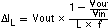Equation 7.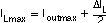with:

f = Switching Frequency (2.25MHz typical)

L = Inductor Value

ΔIL = Peak-to-Peak inductor ripple current

ILmax = Maximum Inductor current

The highest inductor current occurs at maximum Vin.

Open core inductors have a soft saturation characteristic and they can usually handle higher inductor currents versus a comparable shielded inductor.

A more conservative approach is to select the inductor current rating just for the maximum switch current of the corresponding converter. Take into consideration that the core material from inductor to inductor differs and this difference has an impact on the efficiency.

Refer to Table 7 and the typical application circuit examples for possible inductors.

### Table 7. List of Inductors

DIMENSIONS [mm3] INDUCTOR TYPE SUPPLIER
3.2×2.6×1.0 MIPW3226 FDK
3×3×0.9 LPS3010 Coilcraft
2.8×2.6×1.0 VLF3010 TDK
2.8x2.6×1.4 VLF3014 TDK
3×3×1.4 LPS3015 Coilcraft
3.9×3.9×1.7 LPS4018 Coilcraft

#### 9.2.1.2.5.2 Output Capacitor Selection

The advanced fast response voltage mode control scheme of the converters allows the use of tiny ceramic capacitors with a typical value of 10μF to 22μF, without having large output voltage under and overshoots during heavy load transients. Ceramic capacitors with low ESR values results in lowest output voltage ripple, and are therefore recommended. The output capacitor requires either X7R or X5R dielectric. Y5V and Z5U dielectric capacitors are not recommended due to their wide variation in capacitance.

If ceramic output capacitors are used, the capacitor RMS ripple current rating always meets the application requirements. The RMS ripple current is calculated as:

Equation 8.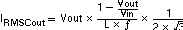At nominal load current the inductive converters operate in PWM mode and the overall output voltage ripple is the sum of the voltage spike caused by the output capacitor ESR, plus the voltage ripple caused by charging and discharging the output capacitor:

Equation 9.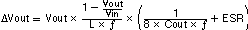Where the highest output voltage ripple occurs at the highest input voltage Vin.

At light load currents the converters operate in Power Save Mode and the output voltage ripple is dependent on the output capacitor value. The output voltage ripple is set by the internal comparator delay and the external capacitor. Higher output capacitors like 22μF values minimize the voltage ripple in PFM Mode and tighten DC output accuracy in PFM Mode.

#### 9.2.1.2.5.3 Input Capacitor Selection

Because of the nature of the buck converter having a pulsating input current, a low ESR input capacitor is required to prevent large voltage transients that can cause misbehavior of the device or interference with other circuits in the system. An input capacitor of 10μF is sufficient.

#### 9.2.1.3 Application Curves

VIN = 3.6 V, and TA = 25 °C, unless otherwise noted.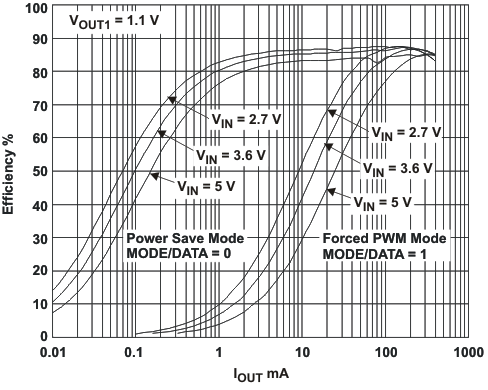A.
Figure 16. Efficiency TPS62401 VOUT1 = 1.1V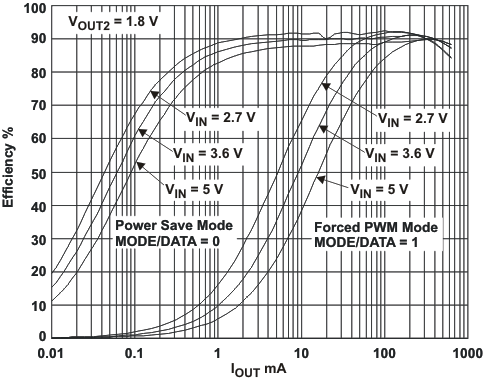A.
Figure 18. Efficiency VOUT 2 = 1.8V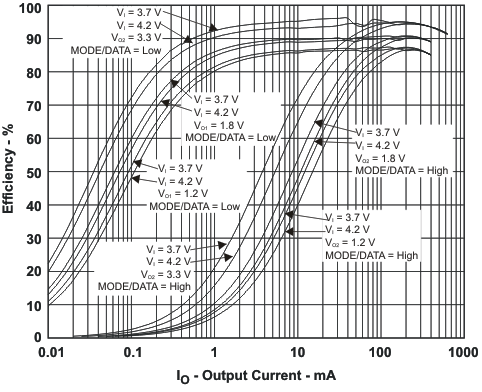A.
Figure 20. Efficiency TPS62402 VOUT1/VOUT2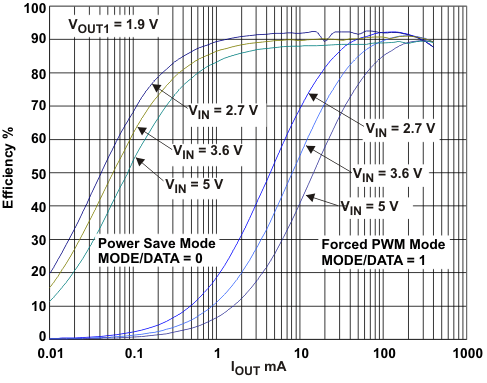A.
Figure 22. Efficiency TPS62404 VOUT1 = 1.9V,
DEF_1 = HIGH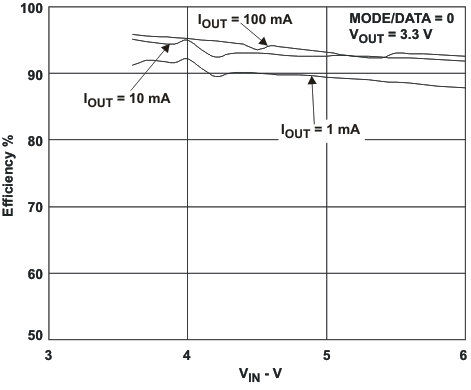A.
Figure 24. EFFICIENCY vs VINA.
Figure 26. DC Output Accuracy VOUT2 = 3.3V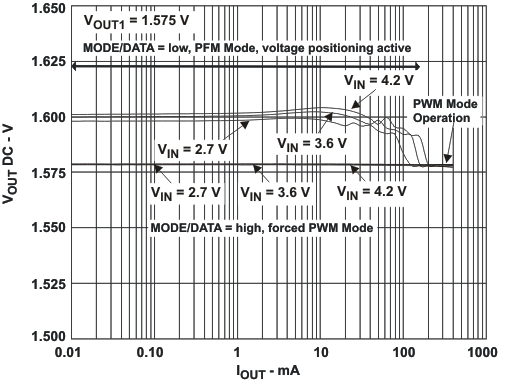A.
Figure 28. DC Output Accuracy VOUT1 = 1.575V,
L = 2.2μH, COUT = 22μF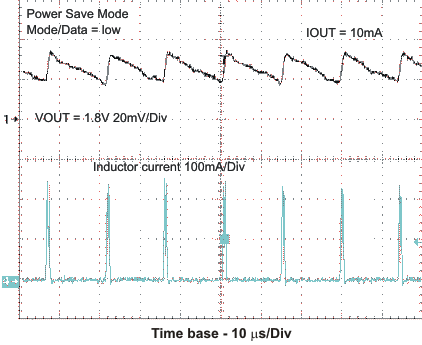Figure 30. Light Load Output Voltage Ripple In Power Save Mode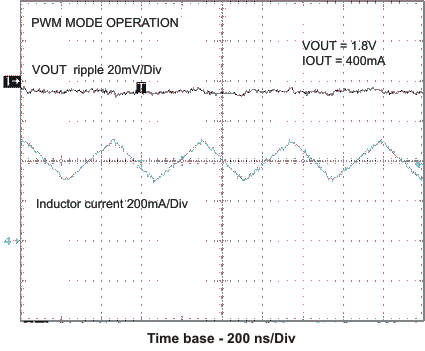Figure 32. Output Voltage Ripple In PWM ModeFigure 34. Load Transient Response PFM/PWM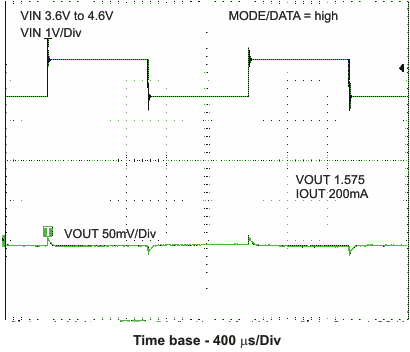Figure 36. Line Transient Response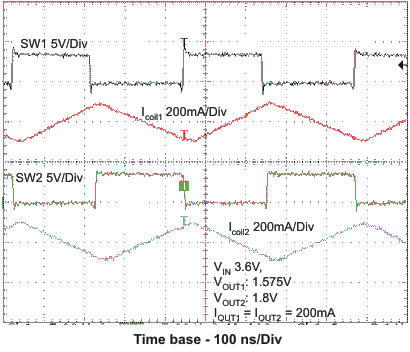Figure 38. Typical Operation VIN = 3.6V,
VOUT1 = 1.575V, VOUT2 = 1.8V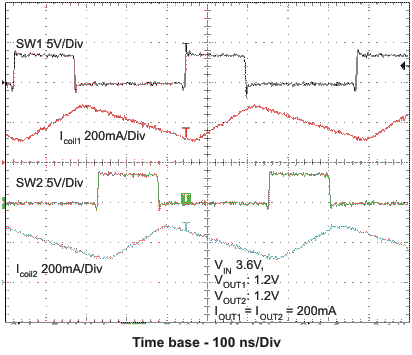Figure 40. Typical Operation VIN = 3.6V,
VOUT1 = 1.2V, VOUT2 = 1.2V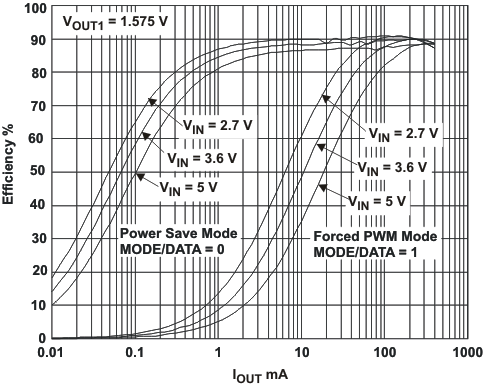A.
Figure 17. Efficiency TPS62401 VOUT1 = 1.575V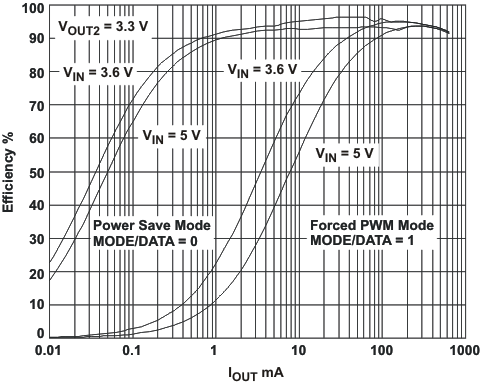A.
Figure 19. Efficiency TPS62400 VOUT 2 = 3.3V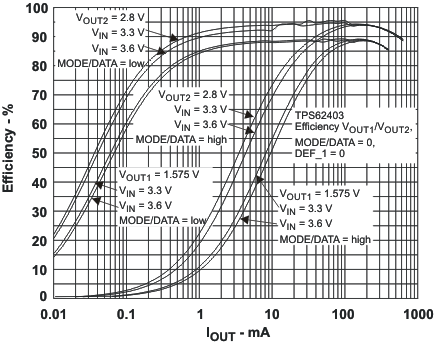A.
Figure 21. Efficiency TPS62403 VOUT1/VOUT2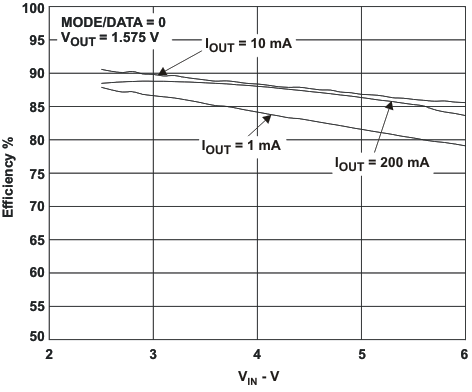A.
Figure 23. Efficiency vs VIN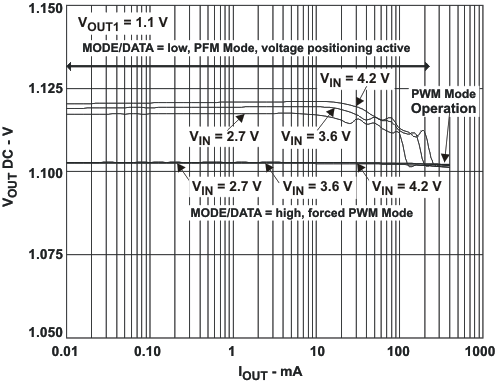A.
Figure 25. DC Output Accuracy VOUT1 = 1.1V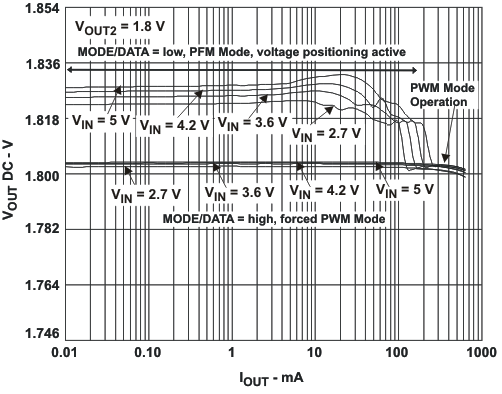A.
Figure 27. DC Output Accuracy VOUT2 = 1.8VA.
Figure 29. DC Output Accuracy VOUT1 = 1.575V,
L = 3.3μH, COUT = 10μF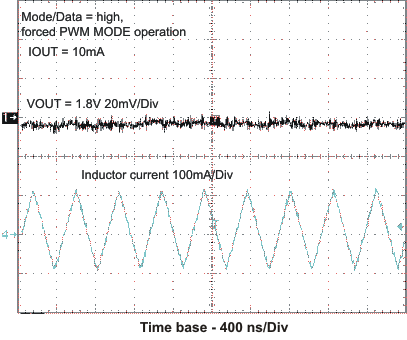Figure 31. Output Voltage Ripple In Forced PWM Mode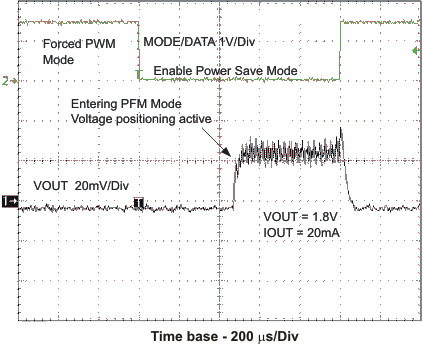Figure 33. Forced PWM/PFM Mode TransitionFigure 35. Load Transient Response PWM Operation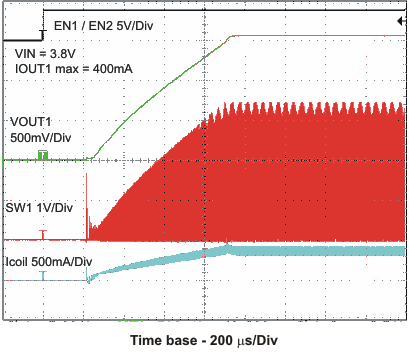Figure 37. Startup Timing One Converter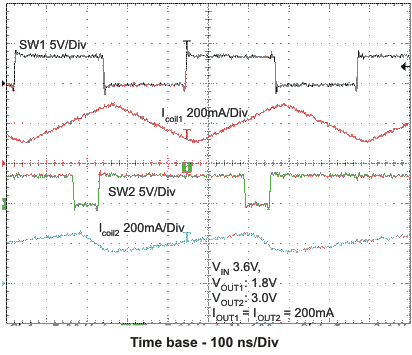Figure 39. Typical Operation VIN = 3.6V,
VOUT1 = 1.8V, VOUT2 = 3.0V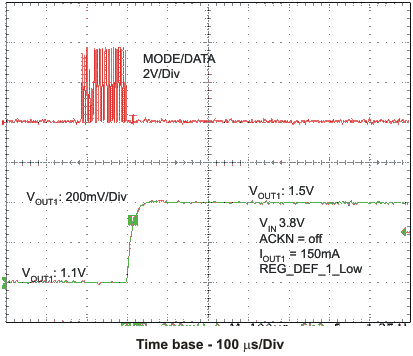Figure 41. VOUT1 Change With Easyscale

#### 9.2.2 Various Output Voltages

The TPS6240x is able to be set for different output voltages. Some examples are shown below.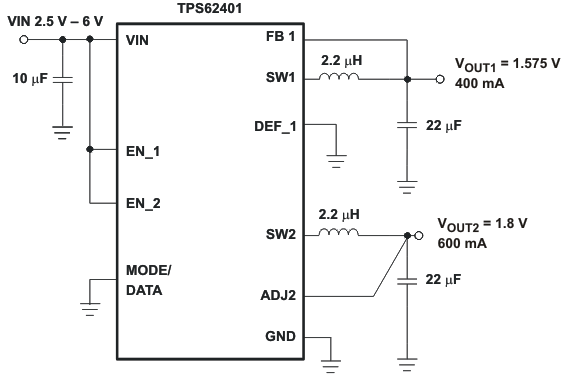Figure 42. TPS62401 Fixed 1.575V/1.8V Outputs, Low PFM Voltage Ripple Optimized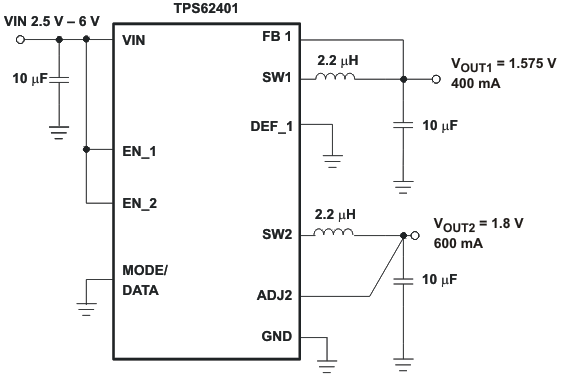Figure 43. TPS62401 Fixed 1.575V/1.8V Outputs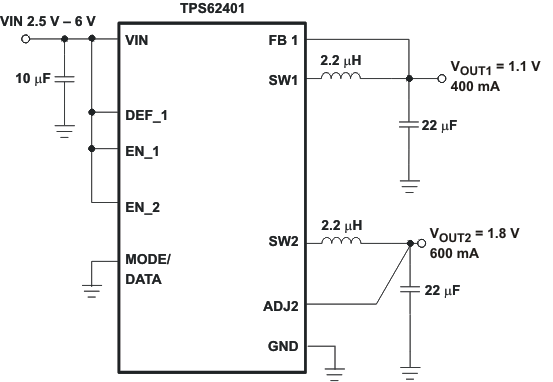Figure 44. TPS62401 Fixed 1.1V/1.8V Outputs, Low PFM Ripple Voltage Optimized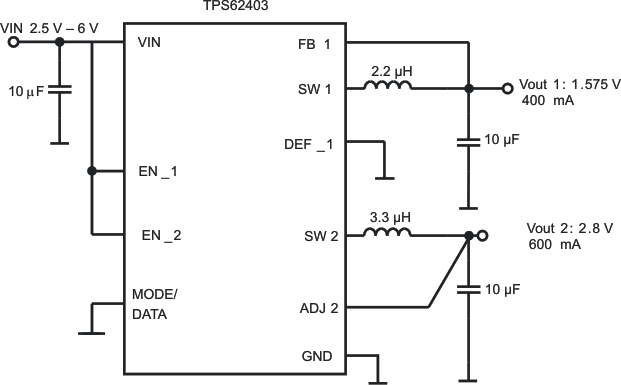Figure 45. TPS62403 1.575V/2.8V Outputs

#### 9.2.2.1 Design Requirements

The TPS6240x step-down converter is set to different output voltages.

#### 9.2.3 Dynamic Voltage Scaling on Converter 1 by DEF_1 Pin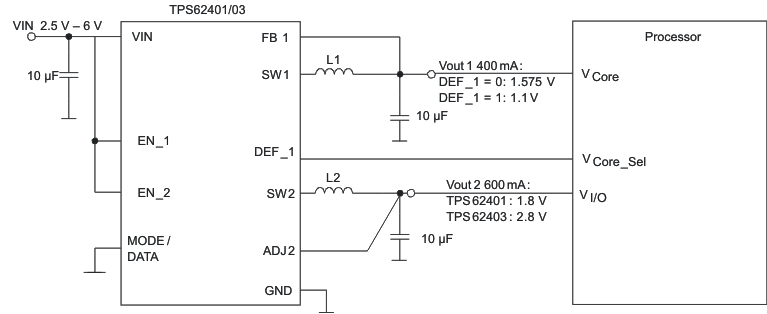Figure 46. Dynamic Voltage Scaling on Converter 1 by DEF_1 Pin

#### 9.2.3.1 Design Requirements

Control the output voltage of the converter 1 through DEF_1 pin by an external processor.

#### 9.2.3.2 Detailed Design Procedure

Connect the DEF_1 pin to the VCore_Sel pin of an external processor, as shown in Figure 46. The processor determines the logic status of the DEF_1 pin which sets the output voltage of the converter 1.

#### Application CurvesFigure 47. TPS62401DEF1_PIN Function For Output Voltage Selection